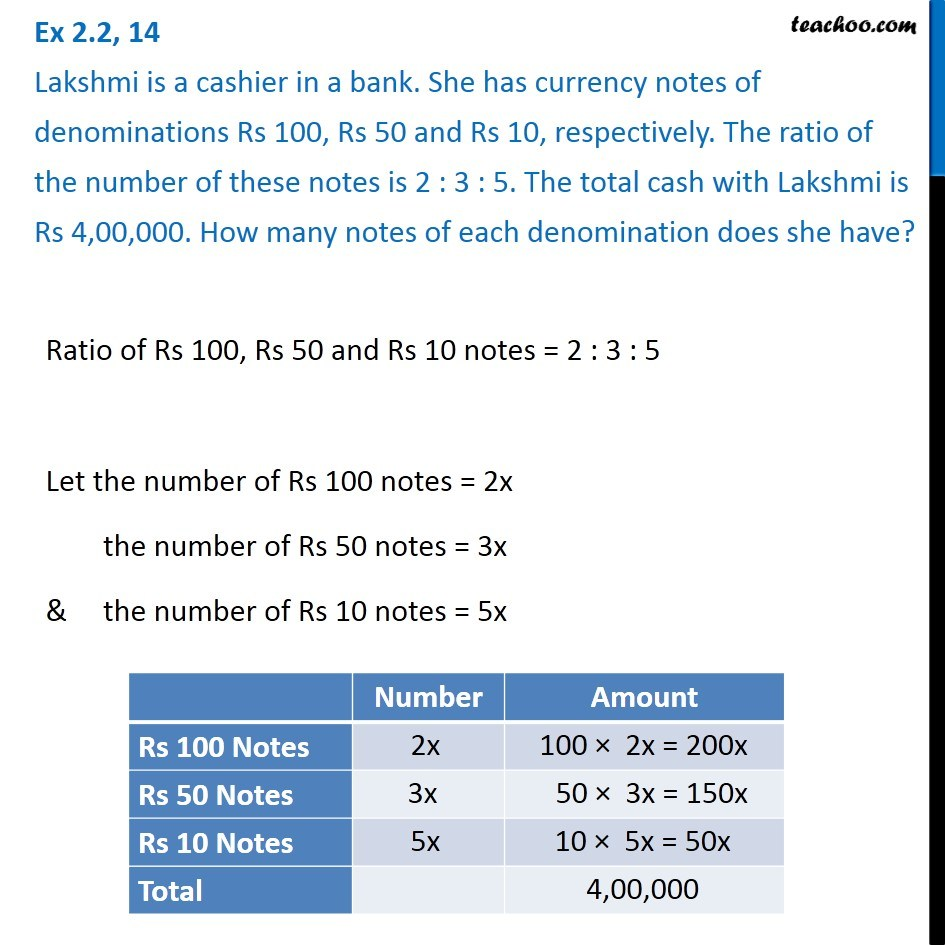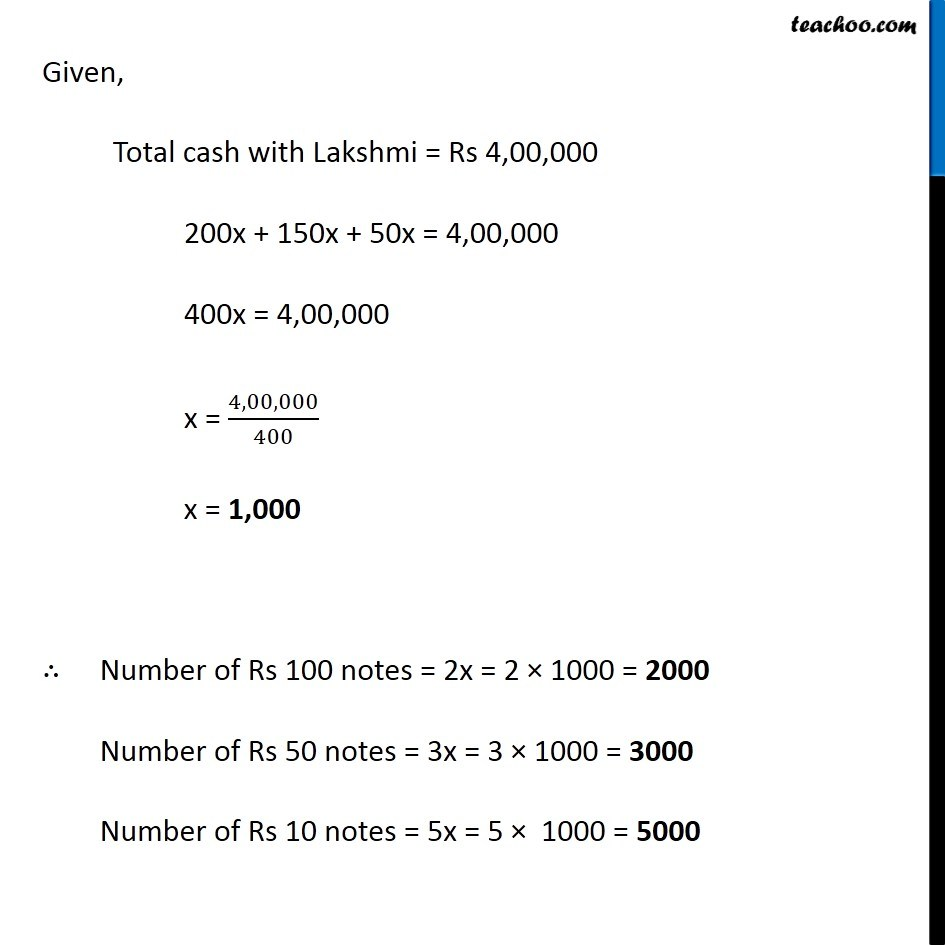Ex 2.2

Chapter 2 Class 8 Linear Equations in One Variable
Serial order wiseGet live Maths 1-on-1 Classs - Class 6 to 12

### Transcript

Ex 2.2, 14 Lakshmi is a cashier in a bank. She has currency notes of denominations Rs 100, Rs 50 and Rs 10, respectively. The ratio of the number of these notes is 2 : 3 : 5. The total cash with Lakshmi is Rs 4,00,000. How many notes of each denomination does she have? Ratio of Rs 100, Rs 50 and Rs 10 notes = 2 : 3 : 5 Let the number of Rs 100 notes = 2x the number of Rs 50 notes = 3x & the number of Rs 10 notes = 5x Given, Total cash with Lakshmi = Rs 4,00,000 200x + 150x + 50x = 4,00,000 400x = 4,00,000 x = 4,00,000/400 x = 1,000 ∴ Number of Rs 100 notes = 2x = 2 × 1000 = 2000 Number of Rs 50 notes = 3x = 3 × 1000 = 3000 Number of Rs 10 notes = 5x = 5 × 1000 = 5000